Next: Discrete variables Up: Building Blocks Previous: Addition and Multiplication

## Gaussian variable with nonlinearity

A nonlinear computation node can be used for constructing nonlinear mappings between the variable nodes. For most nonlinear functions it is impossible to compute the required expectations analytically, but for the function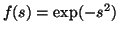the mean and variance have analytical expressions provided that they have Gaussian inputs, i.e. the nonlinearity has to follow immediately after a Gaussian node . The required expectations are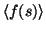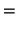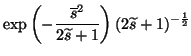(8)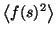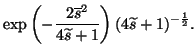(9)

The variance is obtained by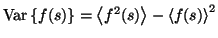. The update of a Gaussian node followed by the nonlinearity is similar to the plain Gaussian node: the gradients of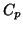w.r.t.and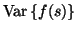are assumed to arise from a quadratic term. This assumption holds since the nonlinearity can only propagate to the mean of Gaussian nodes.

Harri Valpola 2001-10-01